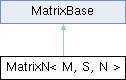MaterialX 1.38.8
Searching...
No Matches
MatrixN< M, S, N > Class Template Reference

The class template for square matrices of scalar values. More...

`#include <Types.h>`

Inheritance diagram for MatrixN< M, S, N >:## Public Types

using RowArray = typename std::array< S, N >

using Iterator = typename std::array< RowArray, N >::iterator

using ConstIterator = typename std::array< RowArray, N >::const_iterator

## Public Member Functions

MatrixN (Uninit)

MatrixN (S s)

MatrixN (const S *begin, const S *end)

Comparison Operators
bool operator== (const M &rhs) const
Return true if the given matrix is identical to this one.

bool operator!= (const M &rhs) const
Return true if the given matrix differs from this one.

bool isEquivalent (const M &rhs, S tolerance) const
Return true if the given matrix is equivalent to this one within a given floating-point tolerance.

Indexing Operators
RowArray & operator[] (size_t i)
Return the row array at the given index.

const RowArray & operator[] (size_t i) const
Return the const row array at the given index.

Component-wise Operators
operator+ (const M &rhs) const

MatrixNoperator+= (const M &rhs)

operator- (const M &rhs) const
Component-wise subtraction of two matrices.

MatrixNoperator-= (const M &rhs)
Component-wise subtraction of two matrices.

operator* (S s) const
Component-wise multiplication of a matrix and a scalar.

MatrixNoperator*= (S s)
Component-wise multiplication of a matrix and a scalar.

operator/ (S s) const
Component-wise division of a matrix by a scalar.

MatrixNoperator/= (S s)
Component-wise division of a matrix by a scalar.

Matrix Algebra
operator* (const M &rhs) const
Compute the matrix product.

MatrixNoperator*= (const M &rhs)
Compute the matrix product.

operator/ (const M &rhs) const
Divide the first matrix by the second (computed as the product of the first matrix and the inverse of the second).

MatrixNoperator/= (const M &rhs)
Divide the first matrix by the second (computed as the product of the first matrix and the inverse of the second).

Iterators
Iterator begin ()

ConstIterator begin () const

Iterator end ()

ConstIterator end () const

Utility
S * data ()
Return a pointer to the underlying data array.

const S * data () const
Return a const pointer to the underlying data array.

## Static Public Member Functions

Static Methods
static constexpr size_t numRows ()
Return the number of rows in this matrix.

static constexpr size_t numColumns ()
Return the number of columns in this matrix.

## Protected Attributes

std::array< RowArray, N > _arr

## Detailed Description

template<class M, class S, size_t N>
class MatrixN< M, S, N >

The class template for square matrices of scalar values.

Inherited by Matrix33 and Matrix44.

The elements of a MatrixN are stored in row-major order, and may be accessed using the syntax `matrix[row][column]`.

Template parameter M is the matrix subclass, S is the scalar element type, and N is the number of rows and columns in the matrix.

The documentation for this class was generated from the following file: